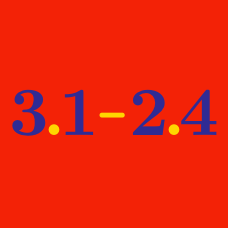Everyday Math

# Decimals: Level 2 Challenges

If the following infinite series $S$ is evaluated as a decimal,what is the 37th digit to the right of the decimal place?

$\large S=\frac { 1 }{ 9 } +\frac { 1 }{ 99 } +\frac { 1 }{ 999 } +\ldots + \frac { 1 }{ { 10 }^{ n }-1 } + \ldots$

Which of the following is equal to

$0.123 \, 123 \, 123 \, 123 \, \ldots ?$

Without actual division find out which of the following rational numbers has a terminating decimal expansion

P)$\frac { 17 }{ 90 }$

Q)$\frac { 33 }{ 50 }$

R)$\frac { 121 }{ { 2 }^{ 2 }\times { 5 }^{ 3 } }$

S)$\frac { 121 }{ { 2 }^{ 3 }\times { 3 }^{ 2 }\times { 7 }^{ 5 } }$

True or False:

$1 > 0.99999\ldots$

$0.20\overline{16}=0.2016161616\ldots =\frac{a}{b}$

Given that $a$ and $b$ are coprime positive integers, find $a+b$.

×